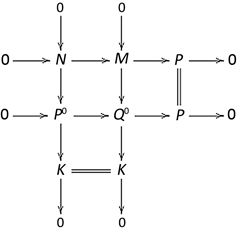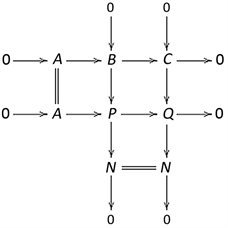# 强泛Gorenstein FC-投射模Strongly Universal Gorenstein FC-Projective Modules

DOI: 10.12677/PM.2021.114078, PDF, HTML, XML, 下载: 21  浏览: 73  国家自然科学基金支持

Abstract: Weak Gorenstein FC-projective and Strongly universal Gorenstein FC-projective modules are in-troduced, the homological properties of the two types of modules are investigated. It is proved that on the right cocoherent ring R, if r.FC.gl.dim(R)<∞, then the class of FC-projective modules, the class of Gorenstein FC-projective modules, the class of weak Gorenstein FC-projective modules, the class of strongly Gorenstein FC-projective modules and the class of strongly universal Gorenstein FC-projective modules are the same class.

1. 引言

1995年，Enochs等人在一般环上引入Gorenstein投(内)射模的概念 。称投射右R-模的正合列 $P=\cdots \to {P}_{1}\to {P}_{0}\to {P}_{-1}\to {P}_{-2}\to \cdots$ 是完全投射分解，如果对任意投射右R-模Q，序列 ${\mathrm{Hom}}_{R}\left(P,Q\right)$ 正合。称右R-模M是Gorenstein投射模，如果存在一个完全投射分解 $P$ 使得 $M\cong$ $\text{Ker}\left({P}_{-1}\to {P}_{-2}\right)$。Gorenstein内射模的定义可对偶给出。2007年，Bennis等人引入强Gorenstein投(内)射模的概念，证明了一个模是Gorenstein投(内)射模当且仅当它是一个强Gorenstein投(内)射模的直和项 。称投射右R-模的正合列 $P=\cdots \to P\stackrel{f}{\to }P\stackrel{f}{\to }P\to \cdots$ 是强完全投射分解，如果对任意投射右R-模Q，序列 ${\mathrm{Hom}}_{R}\left(P,Q\right)$ 正合。称右R-模M是强Gorenstein投射模，如果存在一个强完全投射分解 $P$ 使得 $M\cong \text{Ker}\left(f\right)$。强Gorenstein内射模的定义可对偶给出。2013年，高增辉引入弱Gorenstein投(内)射模的概念 。称右R-模M是弱Gorenstein投射模，如果存在投射右R-模的正合列 $P=\cdots \to {P}_{1}\to {P}_{0}\to {P}^{0}\to {P}^{1}\to \cdots$，使得 $M\cong \text{Ker}\left({P}^{0}\to {P}^{1}\right)$。此时，称序列 $P$M的弱完全投射分解。弱Gorenstein内射模的定义可对偶给出。2014年，陈文静等人引入弱Gorenstein FP-内射模和强泛Gorenstein FP-内射模的概念，讨论了凝聚环上FP-内射模类、Gorenstein FP-内射模类和弱Gorenstein FP-内射模类三者之间的联系(   )。2020年，王玉等人引入Gorenstein FC-投射模的概念，证明了Gorenstein FC-投射模和FC-投射模的等价性，并利用Gorenstein FC-投射模对右Gorenstein FC-半单环进行了刻画 。

2. 弱Gorenstein FC-投射模

$P=\cdots \to {P}_{1}\to {P}_{0}\to {P}^{0}\to {P}^{1}\to \cdots$

2) $w\text{G-}P\left(R\right)\subseteq w\text{GFC-}P\left(R\right)$

3) 由对称性可知，定义2.1中的正合列 $P$ 中所有同态的像、核和余核都是弱Gorenstein FC-投射R-模；

4) 由文献( ，命题2.2)可知， $w\text{GFC-}P\left(R\right)$ 关于直和封闭。

1) $M\in w\text{GFC-}P\left(R\right)$

2) 存在正合列 $0\to M\to {P}^{0}\to {P}^{1}\to \cdots$，其中 ${P}^{i}\in \text{FC-}P\left(R\right)\left(i\in ℕ\right)$

3) 存在正合列 $0\to M\to P\to N\to 0$，其中 $P\in \text{FC-}P\left(R\right)$$N\in w\text{GFC-}P\left(R\right)$

3) Þ 2)因为 $N\in w\text{GFC-}P\left(R\right)$，所以存在N的弱完全FC-投射分解

$P=\cdots \to {P}_{1}\to {P}_{0}\to {P}^{0}\to {P}^{1}\to \cdots$

${P}^{i}\in \text{FC-}P\left(R\right)\left(i\in ℕ\right)$

2) Þ 1) 任取M的一个投射分解 $\cdots \to {P}_{1}\to {P}_{0}\to M\to 0$，与条件中序列首尾相接就得到M的弱完全FC-投射分解

$P=\cdots \to {P}_{1}\to {P}_{0}\to {P}^{0}\to {P}^{1}\to \cdots$1) R是rGF-半单环；

2) $M\in w\text{GFC-}P\left(R\right)$

3) 若 $M\in w\text{G-}I\left(R\right)$，则 $M\in w\text{GFC-}P\left(R\right)$

4) 若 $M\in \text{G-}I\left(R\right)$，则 $M\in w\text{GFC-}P\left(R\right)$

5) 若 $M\in \text{SG-}I\left(R\right)$，则 $M\in w\text{GFC-}P\left(R\right)$

6) 若 $M\in I\left(R\right)$，则 $M\in w\text{GFC-}P\left(R\right)$

6) Þ 1) 设 $M\in I\left(R\right)$，则由条件可知 $M\in w\text{GFC-}P\left(R\right)$，于是由命题2.3可知存在正合列 $0\to M\to P\to N\to 0$，其中 $P\in \text{FC-}P\left(R\right)$$N\in w\text{GFC-}P\left(R\right)$。因为 $M\in I\left(R\right)$，所以正合列 $0\to M\to P\to N\to 0$ 可裂，故由文献( ，命题2.2)可知 $M\in \text{FC-}P\left(R\right)$，于是由文献( ，命题6.3)可知R是rGF-半单环。

R-模类 $X\left(R\right)$ 是投射可解类，如果 $P\left(R\right)\subseteq X\left(R\right)$，且对任意 $X\left(R\right)$ 中的正合列 $0\to {X}^{\prime }\to X\to {X}^{″}\to 0$，其中 ${X}^{″}\in X\left(R\right)$，则 ${X}^{\prime }\in X\left(R\right)⇔X\in X\left(R\right)$ 。下面我们证明 $w\text{GFC-}P\left(R\right)$ 是投射可解类，并且关于直和项封闭。

Þ) 设 $0\to A\to B\to C\to 0$R-模的正合列，只需证当 $B,C\in w\text{GFC-}P\left(R\right)$ 时， $A\in w\text{GFC-}P\left(R\right)$ 即可。因为 $B\in w\text{GFC-}P\left(R\right)$，所以由命题2.3可知存在正合列 $0\to B\to P\to N\to 0$，其中 $P\in \text{FC-}P\left(R\right)$$N\in w\text{GFC-}P\left(R\right)$。考虑推出图$0\to {G}_{n}\to {G}_{n-1}\to \cdots \to {G}_{0}\to M\to 0$

$0\to {H}_{n}\to {H}_{n-1}\to \cdots \to {H}_{0}\to M\to 0,$

$P=\cdots \to {P}_{1}\stackrel{{d}_{1}}{\to }{P}_{0}\stackrel{{d}_{0}}{\to }{P}_{-1}\stackrel{{d}_{-1}}{\to }{P}_{-2}\stackrel{{d}_{-2}}{\to }\cdots$

3. 强泛Gorenstein FC-投射模

$P=\cdots \to P\stackrel{f}{\to }P\stackrel{f}{\to }P\to \cdots$

$\text{FC-}P\left(R\right)\subseteq \text{SGFC-}P\left(R\right)\subseteq \text{SUGFC-}P\left(R\right)\subseteq w\text{GFC-}P\left(R\right)$

2) 由对称性可知，定义3.1中的正合列 $P$ 中所有同态的像、核和余核都是强泛Gorenstein FC-投射R-模；

3) $\text{SUGFC-}P\left(R\right)$ 关于直和封闭；

1) $M\in \text{SUGFC-}P\left(R\right)$

2) 存在正合列 $0\to M\to P\to P\to \cdots$，其中 $P\in \text{FC-}P\left(R\right)$

3) 存在正合列 $0\to M\to P\to M\to 0$，其中 $P\in \text{FC-}P\left(R\right)$

1) R是rSGF-半单环；

2) $M\in \text{SUGFC-}P\left(R\right)$

3) 若 $M\in \text{SUG-}I\left(R\right)$，则 $M\in \text{SUGFC-}P\left(R\right)$

4) 若 $M\in \text{SG-}I\left(R\right)$，则 $M\in \text{SUGFC-}P\left(R\right)$

5) 若 $M\in I\left(R\right)$，则 $M\in \text{SUGFC-}P\left(R\right)$

$P=\cdots \to {P}_{1}\stackrel{{d}_{1}^{P}}{\to }{P}_{0}\stackrel{{d}_{0}^{P}}{\to }{P}_{-1}\stackrel{{d}_{-1}^{P}}{\to }{P}_{-2}\to \cdots$

$Q=\oplus {\sum }^{m}P=\cdots \to Q=\oplus {P}_{i}\stackrel{\oplus {d}_{i}^{P}}{\to }Q=\oplus {P}_{i}\stackrel{\oplus {d}_{i}^{P}}{\to }Q=\oplus {P}_{i}\stackrel{\oplus {d}_{i}^{P}}{\to }\cdots$

  Enochs, E.E. and Jenda, O.M.G. (1995) Gorenstein Injective and Projective Modules. Mathematische Zeitschrift, 220, 611-633. https://doi.org/10.1007/BF02572634  Bennis, D. and Mahdou, N. (2007) Strongly Gorenstein Pro-jective, Injective and Flat Modules. Journal of Pure and Applied Algebra, 210, 437-445. https://doi.org/10.1016/j.jpaa.2006.10.010  Gao, Z.H. (2013) Weak Gorenstein Projective, Injective and Flat Modules. Journal of Algebra and Its Applications, 12, 3841-3858. https://doi.org/10.1142/S0219498812501654  陈文静, 杨晓燕. 弱Gorenstein FP-内射模[J]. 四川师范大学学报(自然科学版), 2014, 37(4): 477-481.  陈文静, 杨晓燕. 强和强泛Gorenstein FP-内射模[J]. 西南大学学报(自然科学版), 2014, 36(8): 75-78.  Wang, Y. and Zhou, D. (2020) Gorenstein FC-Projective Modules. Journal of Algebra and Its Applications, 19, Article ID: 2050066. https://doi.org/10.1142/S0219498820500668  Holm, H. (2004) Gorenstein Homological Dimensions. Journal of Pure and Applied Algebra, 189, 167-193. https://doi.org/10.1016/j.jpaa.2003.11.007  Zhu, X.S. (2013) Resolving Resolution Dimensions. Algebras and Representation Theory, 16, 1165-1191. https://doi.org/10.1007/s10468-012-9351-5  王玉, 周德旭. 关于强Gorenstein FC-投射模[J]. 福建师范大学学报(自然科学版), 2018, 34(5): 12-18.